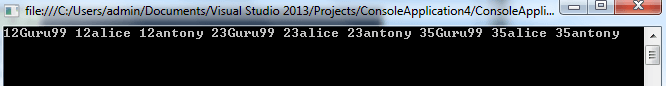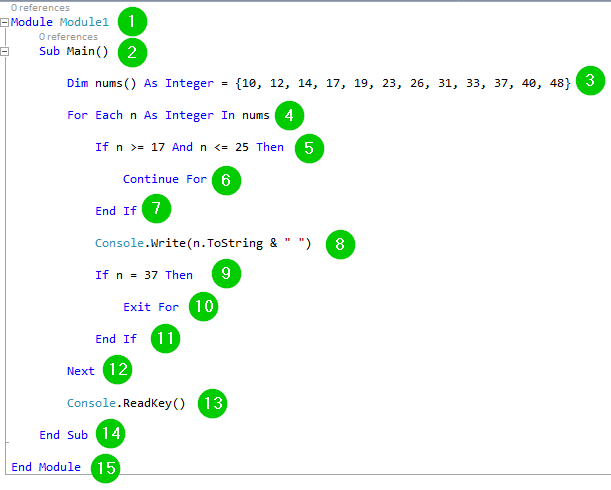# For Each…Next, Exit, Continue Statement in VB.net with EXAMPLE

## What is For Each?

For Each is a Looping command. You should use this statement when you need to repeat a set of statements for every item you have in a collection. You can use it to manipulate all the elements contained in a collection or array.

In this VB. Net tutorial, you will learn,

## Syntax of For Each… Next

The for each statement takes the syntax given below:

```For Each item [ As data_type ] In group
[ statement(s) ]
[ Continue For ]
[ statement(s) ]
[ Exit For ]
[ statement(s) ]
Next [ item ]
```

Here,

• The item is the variable that will be used to iterate over all items of the collection. It is required in the for each statement but optional in the Next statement.
• The data_type is the data type of the element. It is required if the item had not been declared.
• The group is the collection over which statements are to be repeated. It is required.
• The statement(s) is optional. It denotes either one or more statements to be executed for every item in the group.
• The Continue For will transfer control to the beginning of For Each loop. It is optional.
• The Exit For will transfer control out of For Each loop. It is optional.
• The Next marks the end of the For Each loop. It is required.

## Examples

Let us demonstrate how to use this statement:

Step 1) Begin by creating a new console application.

Step 2) Use the following code:

```Module Module1
Sub Main()

Dim myArray() As Integer = {10, 3, 12, 23, 9}

Dim item As Integer

For Each item In myArray

Console.WriteLine(item)

Next

End Sub

End Module
```

Step 3) Click the Start button from the top bar to run the code. You should get the following result:Here is a screenshot of the code:Explanation of Code:

1. Creating a module named Module1.
2. Starting the main sub-procedure.
3. Creating an array named myArray with a set of 4 integers.
4. Creating an integer variable named item.
5. Using the item variable to iterate over the items of the array named myArray.
6. Printing the items found in the array by the above statement on the console.
7. End of the For Each loop.
8. Pausing the console window for a while waiting for a user to take action to close the window.
9. End of the main sub-procedure.
10. End of the module.

## Nested For Loops

The For Each loop can be nested. This will occurs when we put one For Each loop inside another For Each loop. Let us demonstrate this using an example.

Step 1) Create a new console application.

Step 2) Use the following code:

```Module Module1
Sub Main()

Dim nums() As Integer = {12, 23, 35}

Dim names() As String = {"Guru99", "alice", "antony"}

For Each n As Integer In nums

For Each st As String In names

Console.Write(n.ToString & st & " ")

Next

Next

End Sub

End Module
```

Step 3) Click the Start button from the top bar to execute the code. You should get the following output:Here is a screenshot of the code:Explanation of code:

1. Creating a module named Module1.
2. Starting the main sub-procedure.
3. Creating an array named nums with a set of integers.
4. Creating an array named names with a set of names.
5. Creating a variable n and using it to iterate over the items contained in the array named nums. This is the outer For Each loop.
6. Creating a variable named st and using it to iterate over the items contained in the array named names. This is the inner For Each loop.
7. Combining the items from the two arrays. Each item in the nums array will be combined with each item in the names array. The ToString function helps us convert the numbers read from nums array into strings. The ” ” helps us create a space after each combination. The combination has been achieved using the & (ampersand).
8. End of the inner For Each loop.
9. End of the outer For Each loop.
10. Pause the console window waiting for the user to take action to close it.
11. End of the main subprocedure.
12. End of the module.

## Exit For and Continue For

When you use the Exit For statement, the execution will leave the For Each … Next loop and control will be transferred to the statements that come after the Next statement.

When you use the Continue For statement, control will be transferred to the next iteration of your loop. Let us demonstrate this using an example:

Step 1) Begin by creating a new console application.

Step 2) Use the following code:

```Module Module1
Sub Main()

Dim nums() As Integer =
{10, 12, 14, 17, 19, 23, 26, 31, 33, 37, 40, 48}

For Each n As Integer In nums

If n >= 17 And n <= 25 Then
Continue For
End If

Console.Write(n.ToString & " ")

If n = 37 Then
Exit For
End If
Next

End Sub

End Module
```

Step 3) Run the code by clicking the Start button from the top bar. You should get the following result:Here is a screenshot of the code:Explanation of Code:

1. Creating a module named module1.
2. Starting the main sub-procedure.
3. Creating an array named nums with a set of integers.
4. Creating a variable named n then we use it to iterate over the elements contained in the array nums.
5. Using an If…Then condition to check the value of the variable n. If the value is between 17 (17 included) and 25 (25 included), the iteration will skip to the next item in the array.
6. The skipping of the above values is done here. This is the statement necessary for performing the skip. This means that the For Each loop will not run for the skipped items.
7. End the If condition.
8. Printing the values obtained from the array on the console. The ToString function helps us convert the values from numbers to strings. The ” ” will create some space after each printed value.
9. Checking the iteration variable n for when its value is 37 using an If…Then condition.
10. Exiting the For Each loop when the above condition is true, that is, the value of n=37. This means that the iteration on the array items will stop.
11. End of the above If condition.
12. End of the For … Each statement.
13. Pause the console window waiting for the user to take action to close it.
14. End of the main sub-procedure.
15. End of the module.

### Summary

• The For Each statement is used to iterate over every item contained in a collection or an array.
• During development, you can combine For Each statement with the Exit For and Continue For statements to control how the iteration is being done.

#### Top Courses